# The center of gravity G of a 1.5-kg unbalanced tracking wheel is located at a distance r = 18 mm from its geometric center B. The radius of the wheel is R = 60 mm and its centroidal radius of gyration is 44 mm. At the instant shown, the center B of the wheel has a velocity of 0.35 m/s and an acceleration of 1.2 m/s2, both directed to the left. Knowing that the wheel rolls without sliding and neglecting the mass of the driving yoke AB, determine the horizontal force P applied to the yoke.

Question-AnswerCategory: Engineering MechanicsThe center of gravity G of a 1.5-kg unbalanced tracking wheel is located at a distance r = 18 mm from its geometric center B. The radius of the wheel is R = 60 mm and its centroidal radius of gyration is 44 mm. At the instant shown, the center B of the wheel has a velocity of 0.35 m/s and an acceleration of 1.2 m/s2, both directed to the left. Knowing that the wheel rolls without sliding and neglecting the mass of the driving yoke AB, determine the horizontal force P applied to the yoke.

The center of gravity G of a 1.5-kg unbalanced tracking wheel is located at a distance r = 18 mm from its geometric center B. The radius of the wheel is R = 60 mm and its centroidal radius of gyration is 44 mm. At the instant shown, the center B of the wheel has a velocity of 0.35 m/s and an acceleration of 1.2 m/s2, both directed to the left. Knowing that the wheel rolls without sliding and neglecting the mass of the driving yoke AB, determine the horizontal force P applied to the yoke.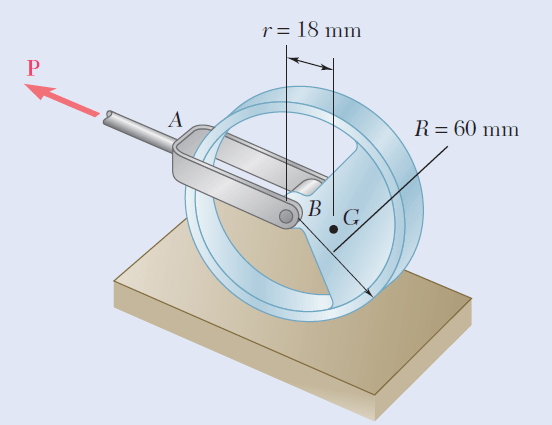Step: 1

Draw the kinematic diagram as shown:Here, consider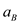and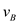are positive towards left.

Step: 2

From the kinematic diagram,Step: 3

Consider the system as follows: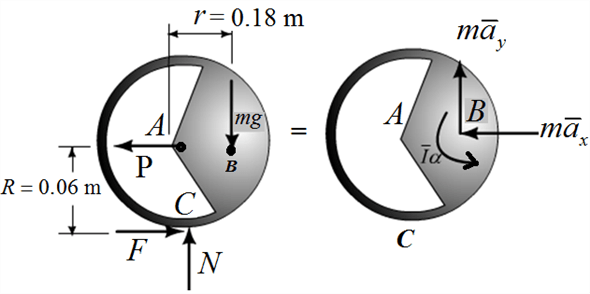Step: 4…… (1)
Here,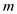is the mass of the wheel,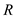is the radius of the wheel,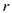is the distance from B to G,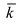is the centroidal radius of gyration of the wheel,is the acceleration of center B, andis the velocity of center B.

Step: 5

Substitute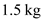forfor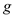forforfor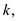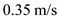for, and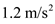forin equation (1).Therefore, the horizontal force P applied to the yoke is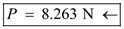.